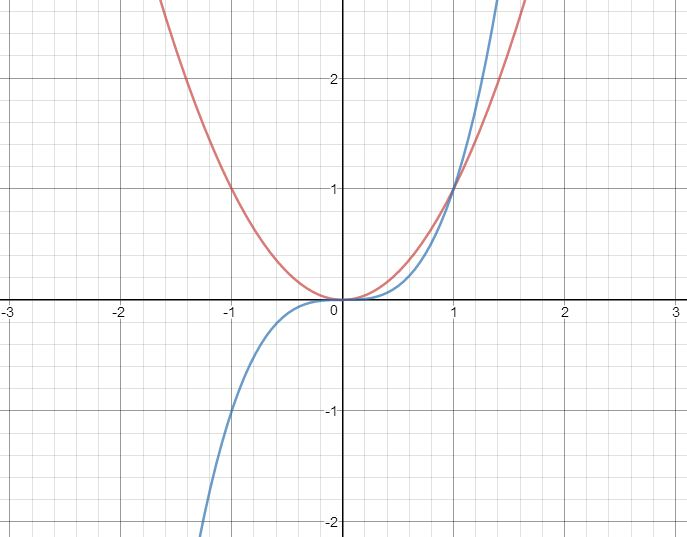# Tangent To Quadratic And Cubic Polynomials

Calculus Level 3The above graph shows the curves of $x^2$ and $x^3$. Clearly, the line $y=0$ is tangent to both the curves. But there exists another such straight line which is tangent to both the curves. Find the equation of the line.

If the line can be represented in the form $ax+by+c=0$, such that $|a|,|b|$ and $|c|$ are positive integers and $\gcd(|a|,|b|,|c|) = 1$, enter your answer as $|a|+|b|+|c|$.

 Notation: $| \cdot |$ denotes the absolute value function.

×